# RS Aggarwal Solutions for Class 9 Maths Chapter 11 - Circles Ex 11A (11.1)

## RS Aggarwal Class 9 RS Aggarwal Solutions for Class 9 MathsChapter 11 - Circles Ex 11A (11.1) Solutions Free PDF

Question 1: In the given figure, O is the centre of the circle and ∠ AOB = 70°  calculate the values of i) ∠ OCA  ii)∠ OAC

Sol.

(i) The angle subtended by an arc of a circle at the center is doubled the angle subtended by the arc at any point on the circumference.

∴ ∠ AOB = 2 × ∠ OCA

⇒ ∠ OCA = 70°/2 = 35° [∵ ∠ AOB =70°]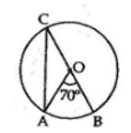(ii) The radius of the circle is

OA = OC

⇒ ∠ OAC = ∠ OCA [∵ base angles of an isosceles triangle are equal]

⇒ ∠ OAC = 35° [as ∠ OCA = 35°]

Question 2:  In the given figure , O is the centre of the circle . If ∠ PBC and ∠ B calculate the values of ∠ ADB.

Sol.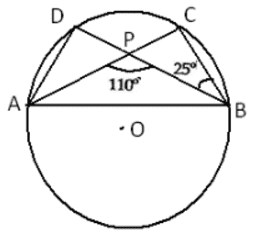It is clear that ∠ ACB = ∠ PCB

Consider the triangle Δ PCB

Applying the sum property, we have

∠ PCB = 180° – (∠ BPC + ∠ PCB)

= 180° – (180° – 110° + 25°)

∠ PCB = 180° – 95° = 85°

Angles in the same segment of a circle are equal.

∴ ∠ ADB = ∠ ACB = 85°

Question 3: In the given figure, O is the centre of the circle.If ∠ ABD = 35° and ∠ BAC = 70°, find ∠ ACB.

Sol.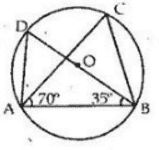It is clear that, BD is the diameter of the circle.

Also we know that, the angle in a semicircle is a right angle.

Now consider the triangle Δ BAD

⇒   ∠ ADB = 180° – (∠ BAD + ∠ABD) [Angle sum property]

⇒      = 180° – (90° + 35°) [∠ BAD = 90° and ∠ABD= 35°]

Angles in the same segment of a circle are equal.

∴ ∠ACB = ∠ ADB = 55°

∴ ∠ACB = 55°

Question 4: In the given figure, O is the centre of the circle. If ∠ACB = 50°, Find ∠OAB .

Sol.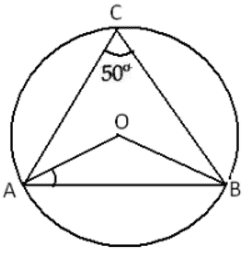The angle subtended by an arc of a circle at the center is doubled the angle subtended by the arc at any point on the circumference.

∴ ∠ AOB = 2 × ∠ ACB

= 2 × 50° [Given]

⇒ ∠AOB = 100° …….(1)

Now, in Δ OAB, we have

OA = OB [radius of the circle]

⇒ ∠ OAB = ∠ OBA [∵ base angles of an isosceles triangle are equal]

Thus we have

∠ OAB = ∠ OBA …….(2)

By angle sum property, we have

Now   ∠AOB + ∠ OAB + ∠ OBA = 180°

⇒ 100° + 2∠ OAB = 180°

⇒ 2∠ OAB = 180° – 100° [from (1) and (2)]

⇒ ∠ OAB = 80°/2 = 40°

∴ ∠ OAB = 40°

Question 5: In the given figure, ∠ABD = 54°  and ∠ABD = 43° . Calculate

i) ∠ACD

ii) ∠BDA

iii) ∠BDA

Sol.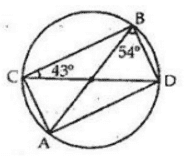(i) Angles in the same segment of a circle are equal.

∠ ABD and ∠ ACD are in the segment AD.

∴ ∠ ACD = ∠ ABD

= 54° [Given]

(ii) Angles in the same segment of a circle are equal.

∠ BAD and ∠ BCD are in the segment BD.

∴ ∠ BAD = ∠ BCD

= 43° [Given]

(iii) Consider the Δ ABD.

By angle sum property, we have

⇒ 43° + ∠ ADB + 54° = 180°

⇒ ∠ ADB = 180° -97° = 83°

⇒ ∠ BDA = 83°

Question 6: In the adjoining figure ,DE is the chord parallel to diameter AC of the circle with Centre O. If ∠ CBD = 60°, calculate ∠ CDE.

Sol.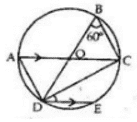Angles in the same segment of a circle are equal.

∴   ∠ CAD =∠ CBD = 60° [Given]

We know that an angle in a semi-circle is a right angle.

∴   ∠ ADC = 90° [angle in a semi-circle]

= 180° – (90° + 60°)

= 180° -150° =30°

∴   ∠ CDE = ∠ ADC =30°

Question 7: In the adjoining figure, O is the centre of the circle. Chord CD is parallel to diameter AB. If∠ ABC = 25°, calculate ∠ CED.

Sol.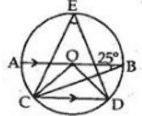Join CO and DO, ∠ BCD = ∠ ABC = 25° [alternate interior angles]

The angle subtended by an arc of a circle at the centre is double the angle subtended by the arc at any point on the circumference.

∴   ∠BOD = 2∠ BCD

= 50° [∠ BCD =25°]

Similarly,

∠AOC = 2∠ ABC

= 50°

AB is a straight line passing through the center.

∴    ∠AOC + ∠COD + ∠BOD = 180°

⇒ 50° + ∠ COD + 50° = 180°

⇒ ∠ COD = 180° – 100° = 80°

∴ ∠ CED = ½ ∠ COD

= 80°/2 = 40°

∴ ∠ CED = 40°

Question 8: In the given figure AB and CD are straight lines to the centre O of a circle .If ∠ AOC= 80° and ∠ CDE = 40° find

I – ∠ DCE

II- ∠ ABC

Sol.

(i) ∠ CED = 90°

In CED, We have

∠ CED + ∠ EDC + ∠ DCE =180°

⇒ 90° + 50° + ∠ DCE = 180°

∴ ∠ DCE = 180° -130°

∠ DCE = 50°   ….(1)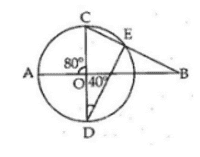(ii) ∠ AOC and ∠ BOC are linear Pair.

∴ ∠ BOC = (180° – 80°) = 100° ….(2)

∴ ∠ABC = 180° – (∠ BOC +∠ DCE)

= 180° – (100° + 50°) [from (1) and (2)]

= 180° – 150° = 30°

Question 9: In the given figure, O is the centre of the circle , ∠ AOB = 40° and ∠ BDC = 100° , find ∠ OBC = 60°

Sol.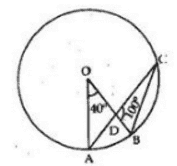The angle subtended by an arc of a circle at the centre is double the angle subtended by the arc at any point on the circumference.

∴   ∠AOB = 2∠ ACB

⇒    = 2∠ ACB [∵ ∠ ACB = ∠ DCB]

DCB=12AOB$\Rightarrow \angle DCB = \frac{1}{2}\angle AOB$

= (12×40)$\left ( \frac{1}{2}\times 40 \right )$ = 20°

Consider the Δ DBC;

By angle sum property, we have

∠ BDC + ∠DCB + ∠ DBC = 180°

⇒ 100° + 20° + ∠DCB = 180°

⇒ ∠ DCB = 180° – 120° = 60°

⇒ ∠ OBC = ∠ DBC = 60°

∴ ∠ OBC = 60°

Question 10: In the adjoining figure ,choprd AC and BD of a circle with centre O , intersect at right angle at E . If  ∠ OAB = 25°, Calculate ∠ EBC

Sol.

Join OB,

∴ ∠ OBA = ∠ OAB = 25° [base angles are equal in isosceles triangle]

Now in Δ OAB, WE have

⇒ ∠OAB + ∠ OBA + ∠ AOB = 180°

⇒  25 + 25 + ∠AOB =180°

⇒  ∠AOB = 180° – 50° = 130°

The angle subtended by an arc of a circle at the centre is double the angle subtended by the arc at any point on the circumference.

∴ ∠ AOB = 2∠ ACB

⇒  ∠ACB = ½ ∠ AOB = ½ × 130° = 65°

⇒  ∠ECB = 65°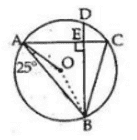In rt  angled Δ BEC,

We know that sum of three angles in a triangle is 180°.

⇒  ∠EBC + ∠BEC +∠ECB = 180°

⇒ ∠EBC + 90° + 65° = 180°

⇒ ∠EBC = 180° – 155° =25°

∴ ∠EBC =25°

Question 11:In the fgiven figure , O is the centre of the circle in which ∠ OAB = 20° and ∠ OCB = 55° . Find

1. ∠ BOC
2. ∠ AOC

Sol.

⇒ ∠ OBC = ∠ OCB =55° [base angles are equal in isosceles triangle]

Consider the triangle Δ BOC.

By angle sum property, we have

∠ BOC = 180° – (∠ OCB + ∠ OBC)

= 180° – (55° + 55°)

= 180° – 110° = 70°

∴ ∠ BOC = 70°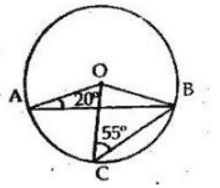Again, OA = OB

⇒ ∠ OBA = ∠ OAB =20° [base angles are equal in isosceles triangle]

Consider the triangle Δ AOB.

By angle sum property, we have

⇒ ∠ AOB = 180° – (∠ OAB + ∠ OBA)

= 180° – (20° + 20°)

= 180° – 40° = 140°

∴ ∠ AOC = ∠ AOB + ∠ BOC

= 140° – 70° = 70°

∴ ∠ AOC = 70°

Question 12: In the given figure , ∠ OAB = 25°. Show that BC is equal to the radius of the circumcircle of Δ ABC whose centre is O.

Sol.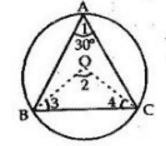Join OB and OC.

The angle subtended by an arc of a circle at the centre is double the angle subtended by the arc at any point on the circumference.

∴ ∠ BOC = 2∠ BAC

= 2 × 30° [∴∠ BAC = 30°]

= 60° …….(1)

Now Consider the triangle Δ BOC.

⇒ ∠OBC = ∠ OCB …….(2)   [base angles are equal in isosceles triangle]

Now in Δ BOC, we have

⇒  ∠BOC + ∠OBC +∠OCB = 180°

⇒  60° + ∠OBC +∠OCB = 180° [from (1) and (2)]

⇒  2∠ OBC = 180° – 60° = 120°

⇒ ∠ OBC = 120°/2 = 60°

⇒ ∠ OBC = 60° [from (2)]

Thus, we have

∠BOC = ∠OBC = ∠OCB = 60°

So, BOC is an equilateral triangle

⇒ OB = OC = BC

∴   BC is the radius of the circumference.

#### Practise This Question

In a sound wave, compression is the region of low pressure.